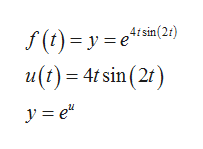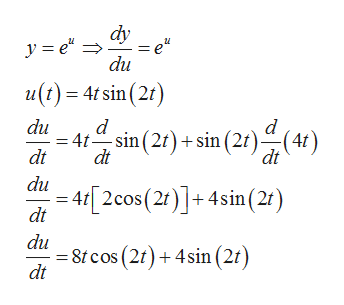Use the chain rule to differentiate f(t)=e^(4t sin(2t))

Question

Use the chain rule to differentiate f(t)=e^(4t sin(2t))

Step 1

Let us take u=4tsin(2t) and f(t)=y, then we have y=e^uhelp_outlineImage Transcriptionclose(t)= y u(t)4 sin (2r ,4/sin(2t ye" fullscreen
Step 2

By chain rule of differentiation , we have

Step 3

Now , we find dy/du and du/dt  by using the result that derivative of e^u with respect to u is e^u  and b...help_outlineImage Transcriptionclosedy y e du u() 4tsin (2 du d - 4t sin (2r)sin (2t)(4f) d dt dt du - 4 [2cos (2)]+ 4sin (2) dt 8cos(2)4sin (2t) dt fullscreen

Want to see the full answer?

See Solution

Want to see this answer and more?

Our solutions are written by experts, many with advanced degrees, and available 24/7

See Solution
Tagged in

Derivative